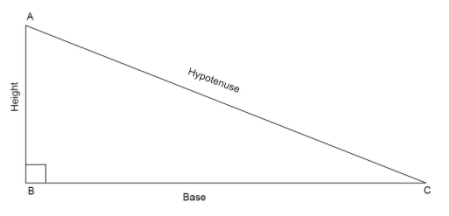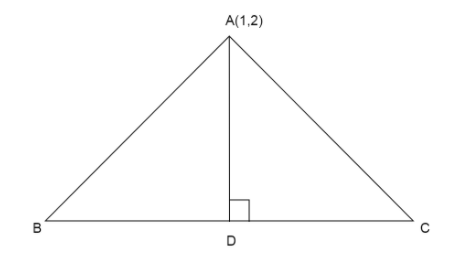QuestionAnswers

# The base of an equilateral triangle is along the line given by $3x + 4y = 9$. If a vertex of the triangle is $(1,2)$ then the length of a side of triangle is A. $\dfrac{{2\sqrt 3 }}{{15}}$B. $\dfrac{{2\sqrt 3 }}{5}$C. $\dfrac{{4\sqrt 3 }}{5}$D. $\dfrac{{4\sqrt 3 }}{{15}}$

Hint: Here we assume length of each side as a and draw a perpendicular from the given vertex to the side which has the equation of line given. Then we apply Pythagoras theorem to the half triangle and find the length of the perpendicular. Now using the formula for distance between a point and the line we find the second equation of length of perpendicular and equate the both lengths to find the value of side.
* Pythagoras theorem states that in a right angled triangle, the sum of square of base and square of height is equal to square of the hypotenuse.
If we have a right angled triangle, $\vartriangle ABC$ with right angle, $\angle B = {90^ \circ }$Then using the Pythagoras theorem we can write that $A{C^2} = A{B^2} + B{C^2}$
* Perpendicular drawn from the vertex of an equilateral triangle bisects the side of the triangle.
* The shortest distance from point $({x_1},{y_1})$ to the line $ax + by + c = 0$ is given by
$D = \dfrac{{\left| {a{x_1} + b{y_1} + c} \right|}}{{\sqrt {{a^2} + {b^2}} }}$

We draw an equilateral triangle whose base is along the line $3x + 4y = 9$ and has a vertex $(1,2)$.We know that an equilateral triangle has all sides of equal length, say ‘a’.
Then the perpendicular AD drawn from A to the base will bisect the base.
So, the length $BD + DC = BC$. Since the base is cut in equal halves
$DC + DC = a \\ 2DC = a \\ DC = \dfrac{a}{2} \\$
Now we apply Pythagoras theorem to $\vartriangle ADC$
$\Rightarrow A{C^2} = A{D^2} + D{C^2} \\ \Rightarrow {a^2} = A{D^2} + {\left( {\dfrac{a}{2}} \right)^2} \\$
Shifting the constants to one side of the equation
$\Rightarrow {a^2} - \dfrac{{{a^2}}}{4} = A{D^2}$
Taking LCM on LHS of the equation
$\Rightarrow \dfrac{{4{a^2} - {a^2}}}{4} = A{D^2} \\ \Rightarrow \dfrac{{3{a^2}}}{4} = A{D^2} \\$
Take square root on both sides of the equation
$\Rightarrow \sqrt {\dfrac{{3{a^2}}}{4}} = \sqrt {A{D^2}} \\ \Rightarrow \dfrac{{\sqrt 3 a}}{2} = AD \\$
So the length of perpendicular $AD = \dfrac{{\sqrt 3 a}}{2}$ … (1)
We have point $(1,2)$ and the shortest distance between the point and the line $3x + 4y = 9$ is $AD$.
Using the formula for shortest distance between a point $({x_1},{y_1})$ and a line $ax + by + c = 0$
$D = \dfrac{{\left| {a{x_1} + b{y_1} + c} \right|}}{{\sqrt {{a^2} + {b^2}} }}$
On comparing line with general equation of line we get $a = 3,b = 4,c = - 9$ and ${x_1} = 1,{x_2} = 2$
$\Rightarrow D = \dfrac{{\left| {3 \times 1 + 4 \times 2 - 9} \right|}}{{\sqrt {{3^2} + {4^2}} }}$
Squaring the terms in the denominator
$\Rightarrow D = \dfrac{{\left| {3 + 8 - 9} \right|}}{{\sqrt {9 + 16} }} \\ \Rightarrow D = \dfrac{{\left| {11 - 9} \right|}}{{\sqrt {25} }} \\ \Rightarrow D = \dfrac{{\left| 2 \right|}}{{\sqrt {{5^2}} }} \\$
Cancel square root with square value
$\Rightarrow D = \dfrac{2}{5}$
Now we have the distance from vertex A to base BC
So, $AD = \dfrac{2}{5}$ … (2)
Equating values of AD from equation (1) and (2)
$\Rightarrow \dfrac{{\sqrt 3 a}}{2} = \dfrac{2}{5}$
Cross multiplying the terms
$\Rightarrow \sqrt 3 a \times 5 = 2 \times 2 \\ \Rightarrow 5\sqrt 3 a = 4 \\$
Shift all the values except a to one side
$\Rightarrow a = \dfrac{4}{{5\sqrt 3 }}$
Rationalize by multiplying both numerator and denominator by $\sqrt 3$
$\Rightarrow a = \dfrac{4}{{5\sqrt 3 }} \times \dfrac{{\sqrt 3 }}{{\sqrt 3 }} \\ \Rightarrow a = \dfrac{{4\sqrt 3 }}{{5 \times 3}} \\ \Rightarrow a = \dfrac{{4\sqrt 3 }}{{15}} \\$
So, the length of the side of the equilateral triangle is $\dfrac{{4\sqrt 3 }}{{15}}$.

So, the correct answer is “Option D”.

Note: Students mostly make the mistake of finding the vertex of the triangle by simply substituting the values of x and y in the equation of line, which is wrong.